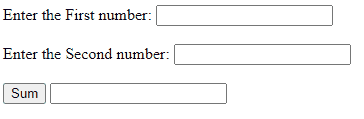GFG App
Open AppBrowser
Continue

Javascript comments explain the code design. They can also be used to prevent the execution of a section of code if necessary. Comments are ignored while the compiler executes the code. Comments are user-friendly as users can get explanations of code using comments.

Syntax:

`// For single line comment`
```/* For block of lines comment
...
...
*/```

Return Value: During the execution of code, comments are ignored.

Example 1: This example illustrates the single-line comment using //.

## Javascript

 `// A single line comment  ` `console.log(``"Hello Geeks!"``);`

Example 2: This example illustrates the multi-line comment using /* … */

## Javascript

 `/* It is multi line comment.  ` `It will not be displayed upon ` `execution of this code */`  `console.log(``"Multiline comment in javascript"``);`

Example 3: Javascript comments can be used to prevent selective execution of code to locate problematic code or while testing new features. This example illustrates that commented code will never execute.

## HTML

 `` `<``html` `lang``=``"en"``>` `<``body``>` `    ``<``label``>Enter the first number` `        ``<``input` `id``=``"num1"``>` `    ``` `    ``<``br``><``br``>` `    ``<``label``>Enter the first number` `        ``<``input` `id``=``"num2"``>` `    ``` `    ``<``br``><``br``>` `    ``<``button` `onclick``=``"add()"``>` `        ``Sum` `    ``` `    ``<``input` `id``=``'sum'``>` `    ``<``script``>` `        ``function add() {` `            ``let x, y, z;` `            ``x = Number(document.getElementById("num1").value);` `            ``y = Number(document.getElementById("num2").value);` `            ``z = x + y;` `            ``// Comment the code section` `            ``//document.getElementById("sum").value = z;` `        ``}` `    ``` `` ``

Output:Explanation: The sum is calculated but not displayed because the code to display the sum is written in a comment and will not be displayed

My Personal Notes arrow_drop_up